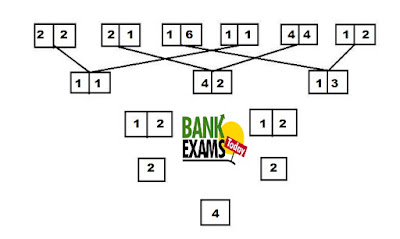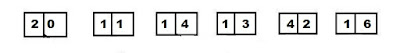Use coupon code TOP15 on all courses

# New Pattern Input/Output Quiz For SBI PO Mains

## Set-1

Direction(1-5): Study the following information carefully and answer the given questions.
A number arrangement machine arranges two digit numbers into a typical manner. Each step takes gives output taking input from the previous step. The following is an illustration of Input and rearrangement. Using the illustration answer the question given below.Input1. If the value “5” is multiply with the final output then what will be the resultant value?
A. 20
B. 16
C. 24
D. 12
E. 28

2. If in the first step the second digit of every number is added and subtracted by 3 then which will be the resultant value?
A. 6
B. 2
C. 1
D. 0
E. 4

3. Which of the following combinations represent the second digit of the third value and the first digit of the second value in Step I of the given input?
A. 3, 2
B. 2, 3
C. 1, 2
D. 2, 1
E. 1, 3

4. Which of the following represents the multiply of the first digit of the second value and the second digit of the first value in Step II of the given input?
A. 4
B. 0
C. 8
D. 32
E.2

5. Which of the following represents the multiply of the first digit of the third value and the second digit of the second value in Step I of the given input?
A. 3
B. 6
C. 2
D. 0
E.9

#### Explanation

Step I:
Multiply the first digit of first number with second digit of fourth Number. Multiply the second digit of first number with first digit fourth number and now value divided by “2”.
Example:-Multiply the first digit  of first number with second digit  of fourth Number. Multiply the second digit  of first number with first digit  fourth number and now value divided by “2”. [2*3=6, 0*1=0 , 60/2=30]

Step II:
Add the first digit of all numbers in Step I and second digit of all numbers in Step I and multiply by “2”. Ex: Add first digit of all numbers [3+1+3] = 7 then multiply by “2” and output is 7*2=14.
Step III:
Divide second digit by first digit

Step IV:## Set-2

Direction (6-10): Study the following information carefully and answer the given questions.
A number arrangement machine arranges two digit numbers into a typical manner. Each step takes gives output taking input from the previous step. The following is an illustration of Input and rearrangement. Using the illustration answer the question given below.Input-6. If the value “6” is added to the final output then what will be the resultant value?
A. 2
B. -14
C. 14
D. -2
E. 4

7. If in the first step the first digit of every number is added and multiply by 3 then which will be the resultant value?
A. 16
B. 24
C. 32
D. 20
E. 22

8. Which of the following combinations represent the second digit of the first value and the first digit of the third value in Step I of the given input?
A. 2, 8
B. 2, 4
C. 4, 2
D. 4, 4
E. 4, 8

9. Which of the following represents the multiply of the second digit of the third value and the second digit of the first value in Step I of the given input?
A. 72
B.32
C. 16
D. 36
E. 08

10. If in the first step the second digit of every number is added and divided by 3 then which will be the resultant value?
A. 7
B. 6
C. 4
D. 2
E.0

#### Explanation

Step I:
Multiply the first digit of first number with second digit of fourth Number. Multiply the second digit of first number with first digit fourth number.

Step II:
Add the first digit of all numbers in Step I and second digit of all numbers in Step I and write down in the reverse order. Ex: Second digit of all numbers [4+9+8] = 21 then the output will be 12

Step III:
Add first digit of value first to with second digit of second value. Add Second digit of value first to with first digit of value second. Ex: [8+1=9] and [0+1=1]

Step IV:
Second number is subtracted from the first number.#### Latest Courses on BankExamsToday1.